# Basic Plotting in R

Created by

Rischan Mafrur

Chonnam National University of South Korea

http://rischanlab.github.io

May 28, 2014

Actually in previous post we already know about how to plot the data into boxplot, histogram, pie chart, matrix scatter plot, so in this page i will show you about more plotting in R

First one is how to plot in 3D plot.

I still use Iris data, in this case i use scatterplot3d library, so you need to install first.

``````library(scatterplot3d)
scatterplot3d(iris\$Petal.Length,iris\$Sepal.Length,iris\$Sepal.Width)
``````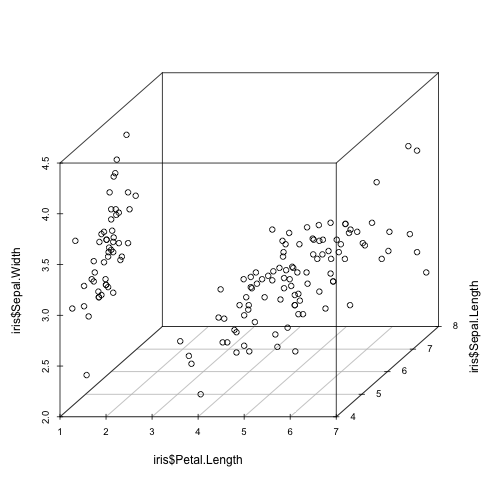Level plot, use library lattice

``````library(lattice)
levelplot(Petal.Width~Sepal.Length*Sepal.Width, iris, cuts=9,
col.regions=grey.colors(10)[10:1])
``````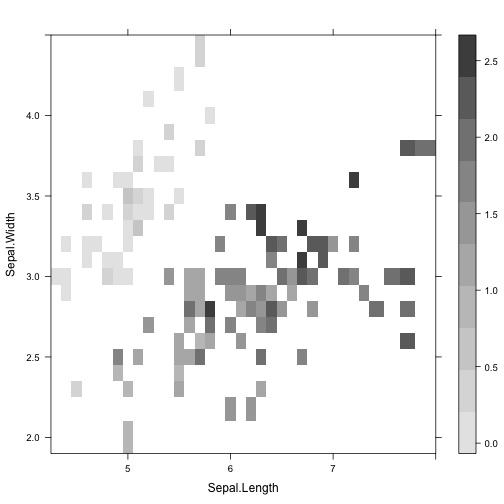with lattice package, we can use contour plot (use volcano dataset)

``````filled.contour(volcano, color=terrain.colors,asp=1,
``````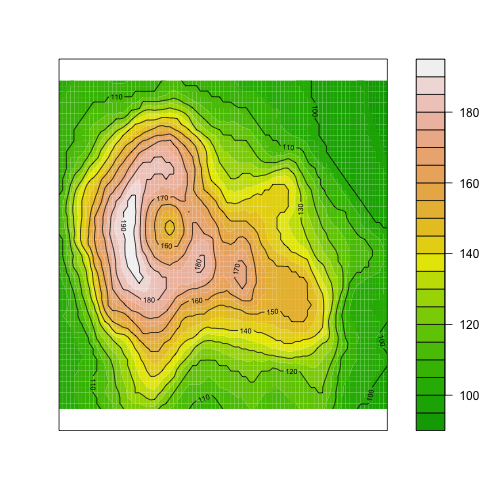``````attributes(volcano)
``````
``````## \$dim
##  87 61
``````
``````str(volcano)
``````
``````##  num [1:87, 1:61] 100 101 102 103 104 105 105 106 107 108 ...
``````

Plotting numeric matrix 3D with persp

``````persp(volcano, theta=25, phi=30, expand=0.5, col="lightblue")
``````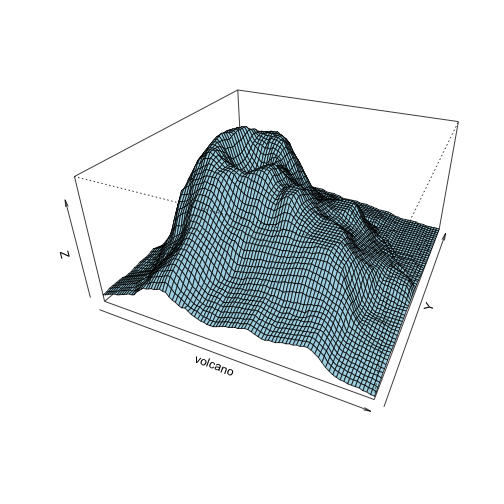Using ggplot, with ggplot we can visualize the data to beautiful plot.

``````library(ggplot2)
p <- ggplot(iris, aes(Sepal.Length, Sepal.Width)) + geom_point(aes(color = Species,
shape = Species))
p + scale_colour_manual(values = c("red", "green", "blue"))
``````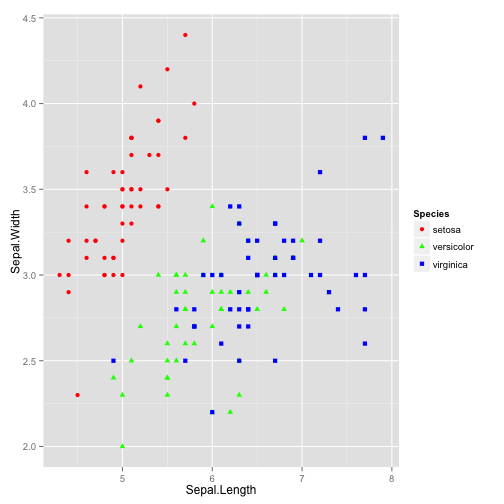``````p + scale_shape_manual(values = c(16, 17, 18))
``````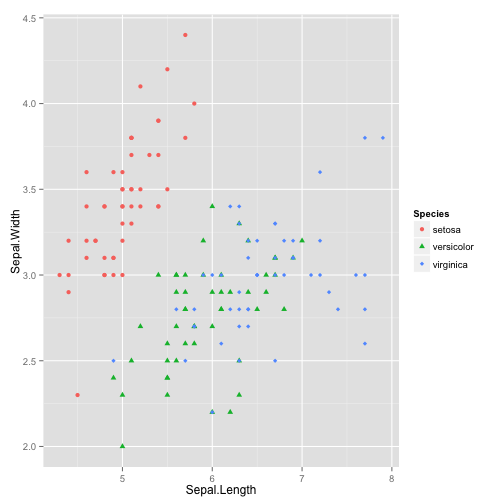for more tutorial about ggplot visit => http://sharpstatistics.co.uk/r/ggplot2-guide/ , http://blog.echen.me/2012/01/17/quick-introduction-to-ggplot2/